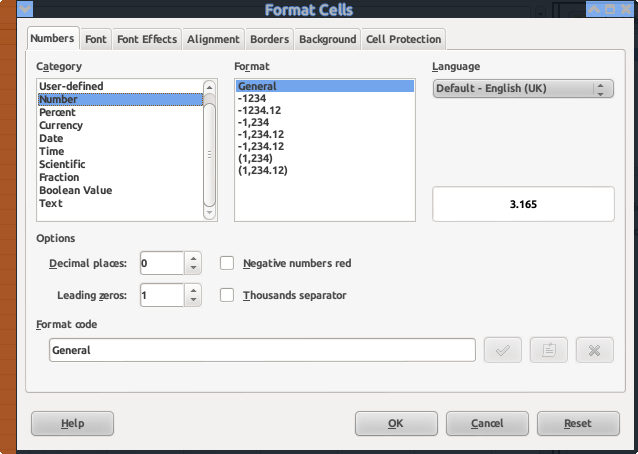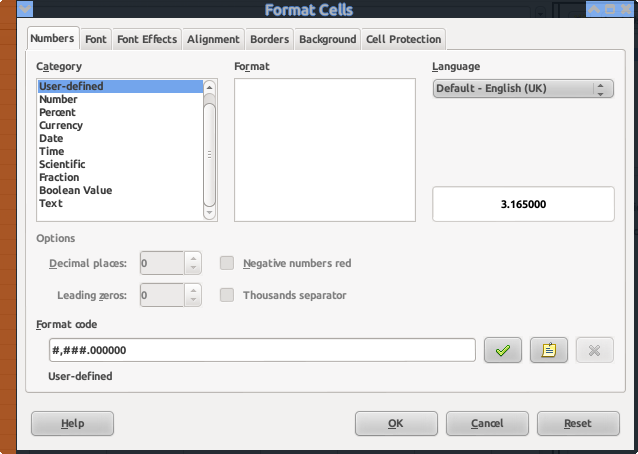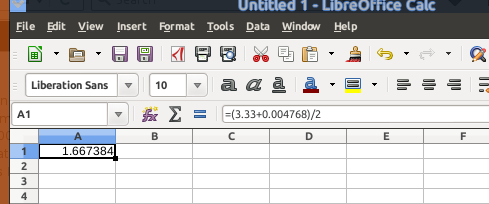# Decimal place precision in calc

LibreOffice ver 4.4.5.2

I am studying various methods of calculating square roots. When I put =(3.33+3)/2 in a cell, the answer is shown as 3.17. I added decimal points to the general number format. This just gave me 3.170000. To 4 decimal places the correct answer is 3.1667 according to the website I am looking at. I tried the answer given in LibreOffice Help, but it doesn’t work. Somehow with the changes I made, it rounds to 3.165000 now.

So here is what I need to do. I can round numbers in my head. So, if I need to, I can go through the mental anguish of rounding 1.667384 to 1.67 if I need to. But, I want the cell to show me 1.667384 when I am doing the calculation. As simple math like I showed (3.33+3)/2 — not having to use a function or something. I’m just wanting to use the cell as a calculator. If this is not possible, I’m sure I can find a calculator on the internet that will allow me long decimal precision. Now I’m going to have to figure out how to get rid of the changes if someone answers this question. Thankfully there are reset or default buttons in the dialogs so I can probably get it back to the way it was.

Check your maths - (3.33 + 3)/2 is exactly 3.165. (6.33/2)

If I put in a new Calc spreadsheet = (3.33 + 3)/2 I get 3.165 without changing any formatting. If I format to 2 decimal places I get 3.17, again correctly rounded up. Any more decimal places gives 3.165 with string of 0’s.

may be: the OP talked about two different samples and wanted to point out his rounding issues in general,
and may be: he just made a very common typo adding 'O’s (letters) instead of '0’s (digits) to the display string ;-), “0,00OOOOO” would show something he worried about …

Hi John Doe

I went through the following steps:

lo-5.0.0.5 (en_GB.UTF-8) Debian 6.0
(brand new Calc sheet)

1. Enter “=(3.33+3)/2” (no quotes)
1. (menu):`Format``Clear Direct Formatting` (`Ctrl+M`)
(from now on we see the default format for the cell)
(in my case, “3.165”)
2. (menu):`Format``Cells...` (`Ctrl+1`)(`Format code` needs to be “0.00” to get your result of “3.17”)
3. Change `Format code` to “#,###.000000” to get 6 decimal place accuracy4. Press `OK`
5. Modify cell formula to “=(3.33+0.004768)/2”
6. Press `OK`The screen now shows the desired result at the desired accuracy. However, the formula used is different to the one that was originally entered.

If this helps then please tick the answer ()

…and/or show you like it with an uptick ()